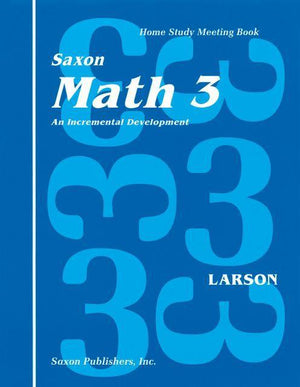# Understanding and practice in math

\$150.00

##### Quantity
- +

Classical Conversations endorses Saxon math as a complete K-12 Curriculum. No curriculum is ever perfect, but Saxon has the history and statistics to prove that it can take a home schooled student through Algebra II and even beyond without the guidance of an expert math teacher.

Math 3 uses simulations and games to help third-grade students understand and practice new concepts. Your child can learn to: skip-count by whole numbers, compare and order numbers, identify ordinal position to the twentieth, identify and complete patterns, add and subtract multi-digit numbers, divide by single-digit divisors, add positive and negative numbers, picture, name, and order fractions, add and subtract fractions with common denominators, understand and calculate measurements, compare and measure mass, identify function rules, graph ordered pairs on a coordinate graph, identify angles, and identify lines of symmetry.

• Includes teacher's manual (spiral bound), student workbook (softcover), and meeting book (softcover)